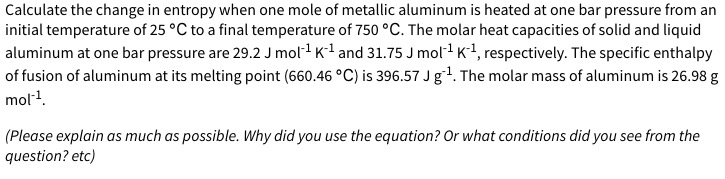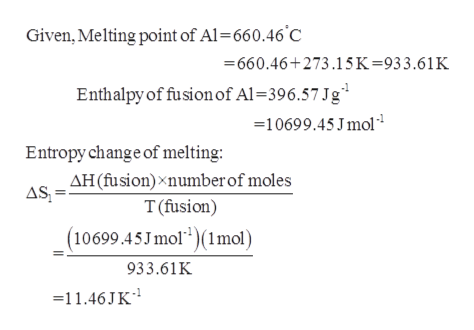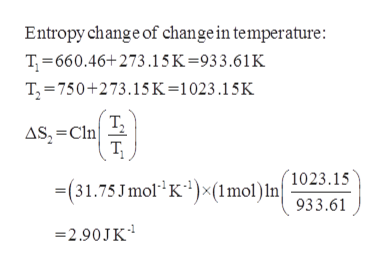# Calculate the change in entropy when one mole of metallic aluminum is heated at one bar pressure from aninitial temperature of 25 °C to a final temperature of 750 °C. The molar heat capacities of solid and liquidaluminum at one bar pressure are 29.2 J mol1 K1 and 31.75 J mol1 K1, respectively. The specific enthalpyof fusion of aluminum at its melting point (660.46 °C) is 396.57 J g1. The molar mass of aluminum is 26.98 gmol1.(Please explain as much as possible. Why did you use the equation? Or what conditions did you see from thequestion? etc)

Question
14 views

Do not copy from others, please!help_outlineImage TranscriptioncloseCalculate the change in entropy when one mole of metallic aluminum is heated at one bar pressure from an initial temperature of 25 °C to a final temperature of 750 °C. The molar heat capacities of solid and liquid aluminum at one bar pressure are 29.2 J mol1 K1 and 31.75 J mol1 K1, respectively. The specific enthalpy of fusion of aluminum at its melting point (660.46 °C) is 396.57 J g1. The molar mass of aluminum is 26.98 g mol1. (Please explain as much as possible. Why did you use the equation? Or what conditions did you see from the question? etc) fullscreen
check_circle

Step 1

The entropy change for melting of aluminum can be calculated ashelp_outlineImage TranscriptioncloseGiven, Melting point of Al-660.46 C 660.46+273.15K=933.61K Enthalpy of fusion of Al = 3 96.57 Jg 10699.45 J mol Entropy change of melting: AH(fusion)xnumber of moles AS T (fusion) (10699.45Jmol2)(1mol) 933.61K -11.46JK fullscreen
Step 2

The entropy change for temperature of...help_outlineImage TranscriptioncloseEntropy change of change in temperature: T 660.46+273 .15K=933.61K T, 750+273.15K=1023 .1 5K Т, AS, =Cln т, (1023 .15 (31.75Jmol K)(1mol) In 933.61 2.90JK fullscreen

### Want to see the full answer?

See Solution

#### Want to see this answer and more?

Solutions are written by subject experts who are available 24/7. Questions are typically answered within 1 hour.*

See Solution
*Response times may vary by subject and question.
Tagged in

### Physical Chemistry